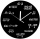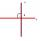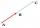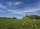# Equation + analytic geometry - math problems

#### Number of problems found: 52

• Parametric equationPoint A [6; -2]. Point B = [-3; 1] Write the parametric equation of the line BA so that t belongs to the closed interval 0; 3
• CircleFrom the equation of a circle: ? Calculate the coordinates of the center of the circle S[x0, y0] and radius of the circle r.
• ParabolaFind the equation of a parabola that contains the points at A[6; -5], B[14; 9], C[23; 6]. (use y = ax2+bx+c)
• Find the 12Find the equation of the circle with center (3,7) and circumference 8π units.
• Equation of circle 2Find the equation of a circle which touches the axis of y at a distance 4 from the origin and cuts off an intercept of length 6 on the axis x.
• Find the 13Find the equation of the circle inscribed in the rhombus ABCD where A[1, -2], B[8, -3] and C[9, 4].4 m long ladder touches the cube 1mx1m at the wall. How high reach on the wall?
• PerpendicularDetermine the slope of the line perpendicular to the line p: y = -x +4.
• Sphere equationObtain the equation of sphere its centre on the line 3x+2z=0=4x-5y and passes through the points (0,-2,-4) and (2,-1,1).
• Equation of circlefind an equation of the circle with indicated properties: a. center at (-3,5), diameter 20. b. center at origin and diameter 16.
• Distance problemA=(x, x) B=(1,4) Distance AB=√5, find x;
• LineWrite an equation of a line parallel to To 9x + 3y = 8 That Passes Through The Point (-1, -4). Write in form ax+by=c.
• Linear independenceDetermine if vectors u=(-4; -5) and v=(20; 25) are linear Linear dependent.
• Circle - AGFind the coordinates of circle and its diameter if its equation is: ?
• Distance problem 2A=(x,2x) B=(2x,1) Distance AB=√2, find value of x
• Find parametersFind parameters of the circle in the plane - coordinates of center and radius: ?
• V - slopeThe slope of the line whose equation is -3x -9 = 0 is
• AngleA straight line p given by the equation ?. Calculate the size of angle in degrees between line p and y-axis.
• CircleWrite the equation of a circle that passes through the point [0,6] and touch the X-axis point [5,0]: ?
• Curve and lineThe equation of a curve C is y=2x² -8x+9 and the equation of a line L is x+ y=3 (1) Find the x co-ordinates of the points of intersection of L and C. (2) Show that one of these points is also the stationary point of C?

Do you have an interesting mathematical word problem that you can't solve it? Submit a math problem, and we can try to solve it.

We will send a solution to your e-mail address. Solved examples are also published here. Please enter the e-mail correctly and check whether you don't have a full mailbox.

Please do not submit problems from current active competitions such as Mathematical Olympiad, correspondence seminars etc...

For Basic calculations in analytic geometry is a helpful line slope calculator. From coordinates of two points in the plane it calculate slope, normal and parametric line equation(s), slope, directional angle, direction vector, the length of segment, intersections the coordinate axes etc. Do you have a linear equation or system of equations and looking for its solution? Or do you have quadratic equation? Equations Math problems. Analytic geometry - math word problems.